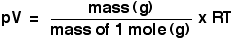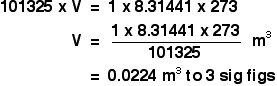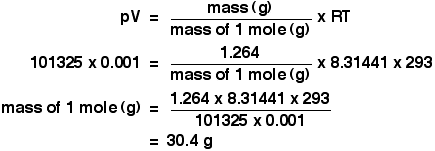IDEAL GASES AND THE IDEAL GAS LAW

```
```

This page looks at the assumptions which are made in the Kinetic Theory about ideal gases, and takes an introductory look at the Ideal Gas Law: pV = nRT. This is intended only as an introduction suitable for chemistry students at about UK A level standard (for 16 - 18 year olds), and so there is no attempt to derive the ideal gas law using physics-style calculations.

```
```

Kinetic Theory assumptions about ideal gases

There is no such thing as an ideal gas, of course, but many gases behave approximately as if they were ideal at ordinary working temperatures and pressures. Real gases are dealt with in more detail on another page.

The assumptions are:

• Gases are made up of molecules which are in constant random motion in straight lines.

• The molecules behave as rigid spheres.

• Pressure is due to collisions between the molecules and the walls of the container.

• All collisions, both between the molecules themselves, and between the molecules and the walls of the container, are perfectly elastic. (That means that there is no loss of kinetic energy during the collision.)

• The temperature of the gas is proportional to the average kinetic energy of the molecules.

And then two absolutely key assumptions, because these are the two most important ways in which real gases differ from ideal gases:

• There are no (or entirely negligible) intermolecular forces between the gas molecules.

• The volume occupied by the molecules themselves is entirely negligible relative to the volume of the container.

```
```

The Ideal Gas Equation

The ideal gas equation is:

pV = nRT

On the whole, this is an easy equation to remember and use. The problems lie almost entirely in the units. I am assuming below that you are working in strict SI units (as you will be if you are doing a UK-based exam, for example).

```
```

Exploring the various terms

Pressure, p

Pressure is measured in pascals, Pa - sometimes expressed as newtons per square metre, N m-2. These mean exactly the same thing.

Be careful if you are given pressures in kPa (kilopascals). For example, 150 kPa is 150000 Pa. You must make that conversion before you use the ideal gas equation.

Should you want to convert from other pressure measurements:

• 1 atmosphere = 101325 Pa

• 1 bar = 100 kPa = 100000 Pa

```
```

Volume, V

This is the most likely place for you to go wrong when you use this equation. That's because the SI unit of volume is the cubic metre, m3 - not cm3 or dm3.

1 m3 = 1000 dm3 = 1 000 000 cm3

So if you are inserting values of volume into the equation, you first have to convert them into cubic metres.

You would have to divide a volume in dm3 by 1000, or in cm3 by a million.

Similarly, if you are working out a volume using the equation, remember to covert the answer in cubic metres into dm3 or cm3 if you need to - this time by multiplying by a 1000 or a million.

If you get this wrong, you are going to end up with a silly answer, out by a factor of a thousand or a million. So it is usually fairly obvious if you have done something wrong, and you can check back again.

```
```

Number of moles, n

This is easy, of course - it is just a number. You already know that you work it out by dividing the mass in grams by the mass of one mole in grams.

You will most often use the ideal gas equation by first making the substitution to give:I don't recommend that you remember the ideal gas equation in this form, but you must be confident that you can convert it into this form.

```
```

The gas constant, R

A value for R will be given you if you need it, or you can look it up in a data source. The SI value for R is 8.31441 J K-1 mol-1.

Note:  You may come across other values for this with different units. A commonly used one in the past was 82.053 cm3 atm K-1 mol-1. The units tell you that the volume would be in cubic centimetres and the pressure in atmospheres. Unfortunately the units in the SI version aren't so obviously helpful.

The temperature, T

The temperature has to be in kelvin. Don't forget to add 273 if you are given a temperature in degrees Celsius.

```
```

Using the ideal gas equation

Calculations using the ideal gas equation are included in my calculations book (see the link at the very bottom of the page), and I can't repeat them here. There are, however, a couple of calculations that I haven't done in the book which give a reasonable idea of how the ideal gas equation works.

The molar volume at stp

If you have done simple calculations from equations, you have probably used the molar volume of a gas.

1 mole of any gas occupies 22.4 dm3 at stp (standard temperature and pressure, taken as 0°C and 1 atmosphere pressure). You may also have used a value of 24.0 dm3 at room temperature and pressure (taken as about 20°C and 1 atmosphere).

These figures are actually only true for an ideal gas, and we'll have a look at where they come from.

```
```

We can use the ideal gas equation to calculate the volume of 1 mole of an ideal gas at 0°C and 1 atmosphere pressure.

First, we have to get the units right.

0°C is 273 K. T = 273 K

1 atmosphere = 101325 Pa. p = 101325 Pa

We know that n = 1, because we are trying to calculate the volume of 1 mole of gas.

And, finally, R = 8.31441 J K-1 mol-1.

Slotting all of this into the ideal gas equation and then rearranging it gives:And finally, because we are interested in the volume in cubic decimetres, you have to remember to multiply this by 1000 to convert from cubic metres into cubic decimetres.

The molar volume of an ideal gas is therefore 22.4 dm3 at stp.

And, of course, you could redo this calculation to find the volume of 1 mole of an ideal gas at room temperature and pressure - or any other temperature and pressure.

```
```

Finding the relative formula mass of a gas from its density

This is about as tricky as it gets using the ideal gas equation.

The density of ethane is 1.264 g dm-3 at 20°C and 1 atmosphere. Calculate the relative formula mass of ethane.

The density value means that 1 dm3 of ethane weighs 1.264 g.

Again, before we do anything else, get the awkward units sorted out.

A pressure of 1 atmosphere is 101325 Pa.

The volume of 1 dm3 has to be converted to cubic metres, by dividing by 1000. We have a volume of 0.001 m3.

The temperature is 293 K.

Now put all the numbers into the form of the ideal gas equation which lets you work with masses, and rearrange it to work out the mass of 1 mole.The mass of 1 mole of anything is simply the relative formula mass in grams.

So the relative formula mass of ethane is 30.4, to 3 sig figs.

```
```

Now, if you add up the relative formula mass of ethane, C2H6 using accurate values of relative atomic masses, you get an answer of 30.07 to 4 significant figures. Which is different from our answer - so what's wrong?

There are two possibilities.

• The density value I have used may not be correct. I did the sum again using a slightly different value quoted at a different temperature from another source. This time I got an answer of 30.3. So the density values may not be entirely accurate, but they are both giving much the same sort of answer.

• Ethane isn't an ideal gas. Well, of course it isn't an ideal gas - there's no such thing! However, assuming that the density values are close to correct, the error is within 1% of what you would expect. So although ethane isn't exactly behaving like an ideal gas, it isn't far off.

If you need to know about real gases, now is a good time to read about them.

```
```
 Questions to test your understanding If this is the first set of questions you have done, please read the introductory page before you start. You will need to use the BACK BUTTON on your browser to come back here afterwards. questions on ideal gases answers
```
```

Where would you like to go now?

To explore real gases . . .

To the kinetic theory menu . . .

To the Physical Chemistry menu . . .

To Main Menu . . .

```
```